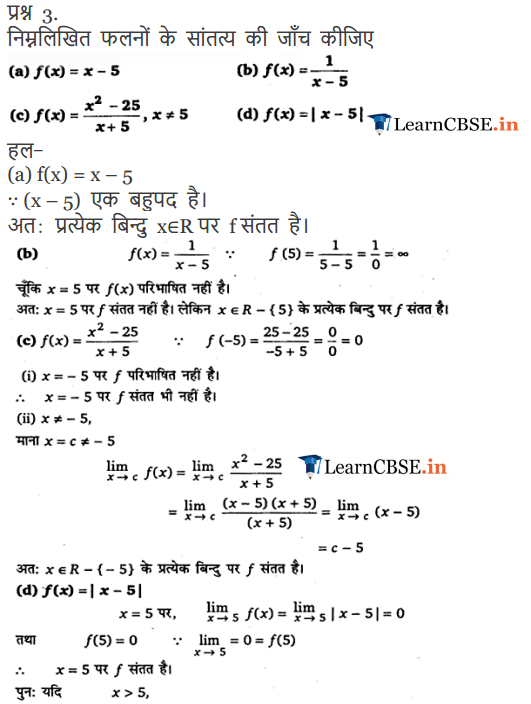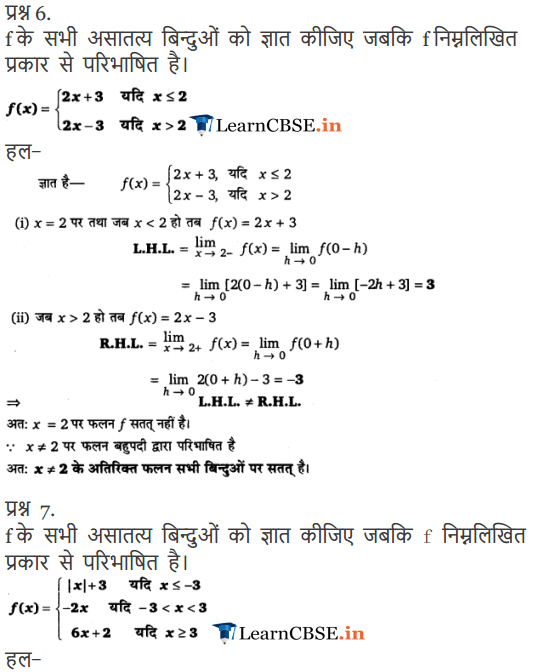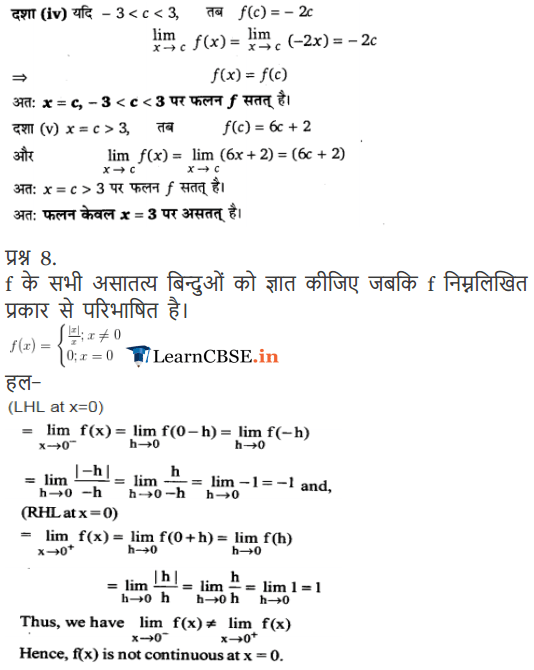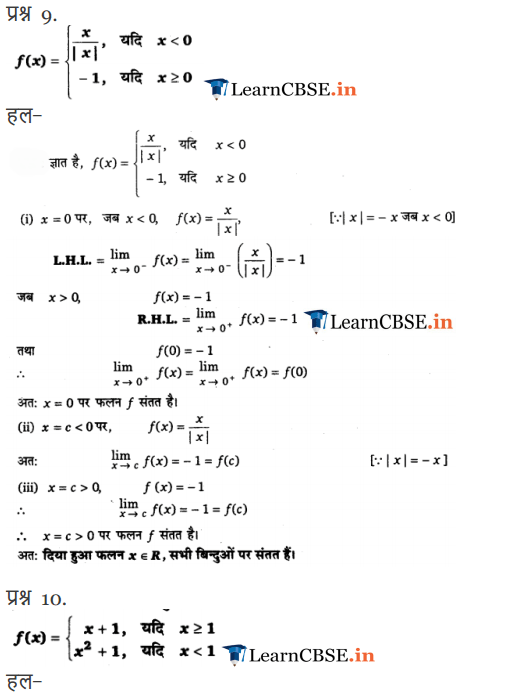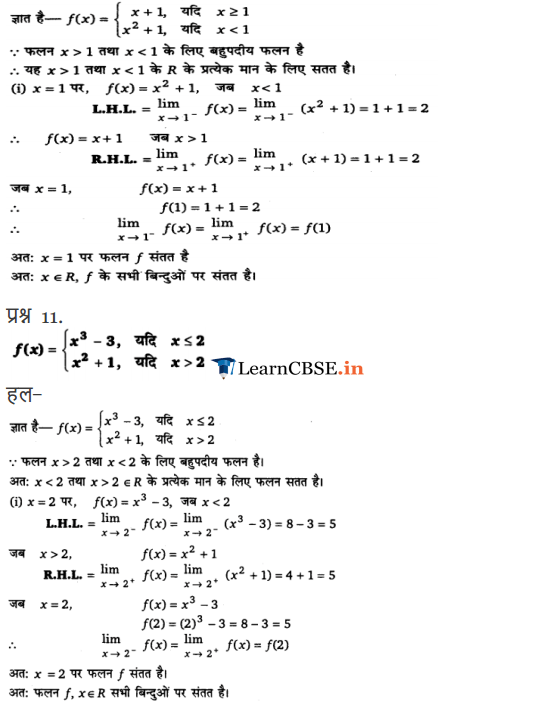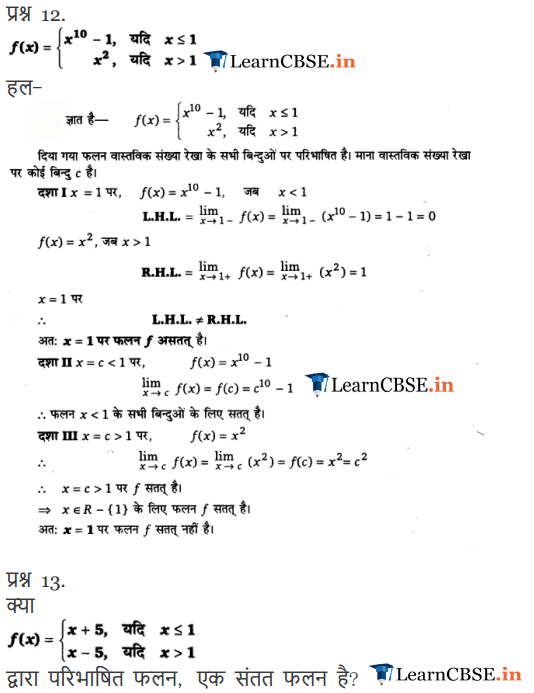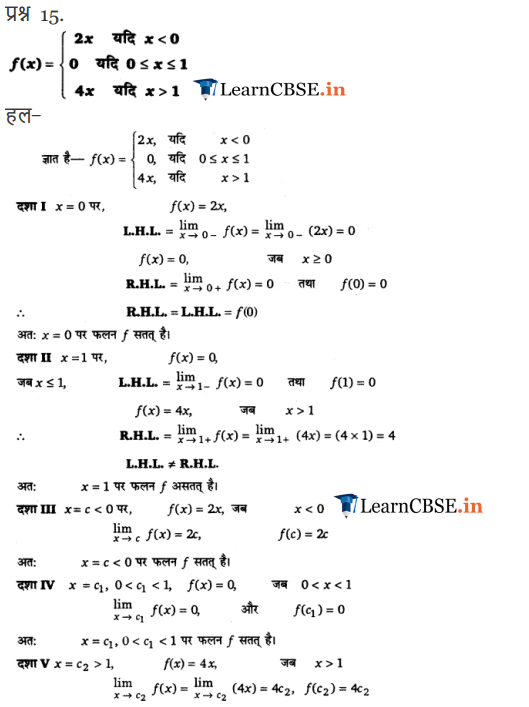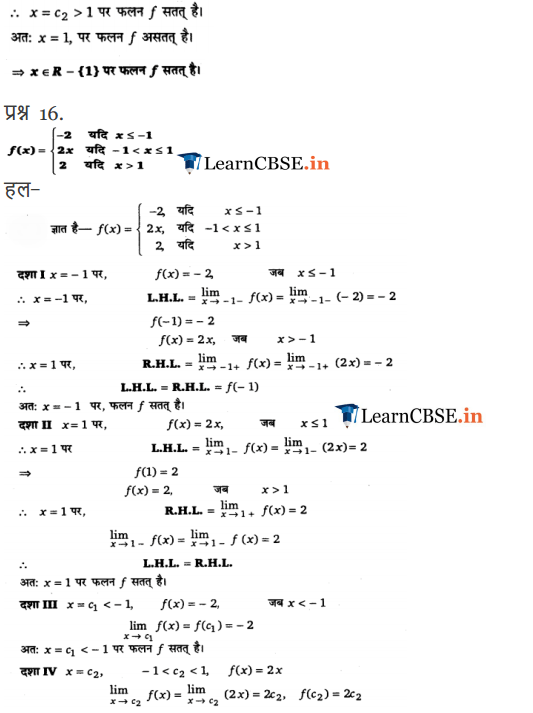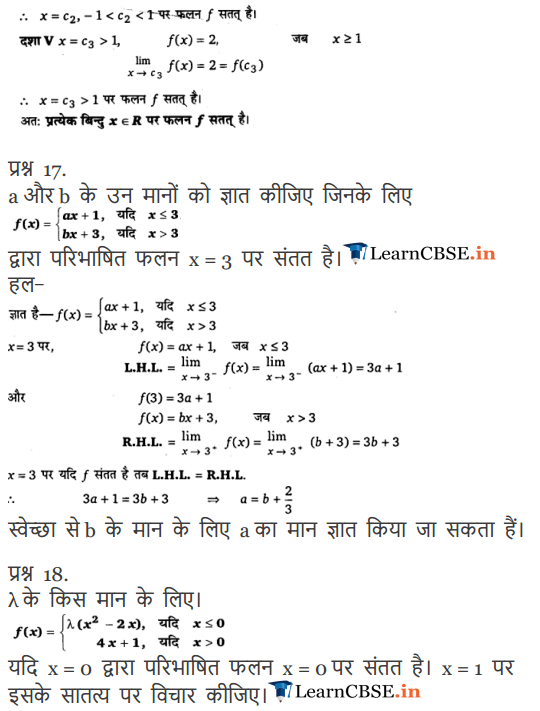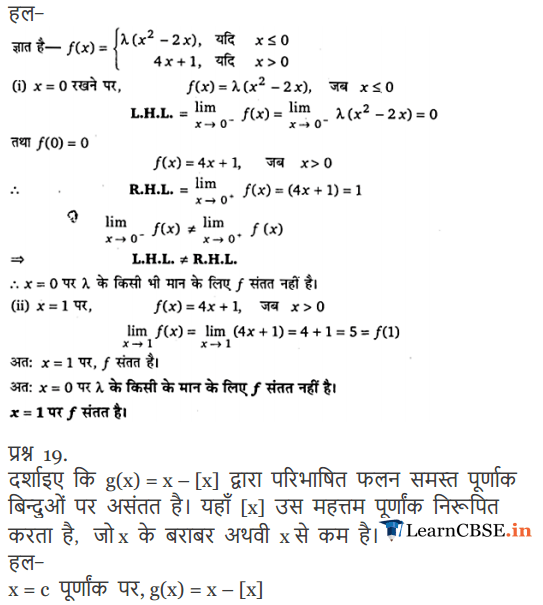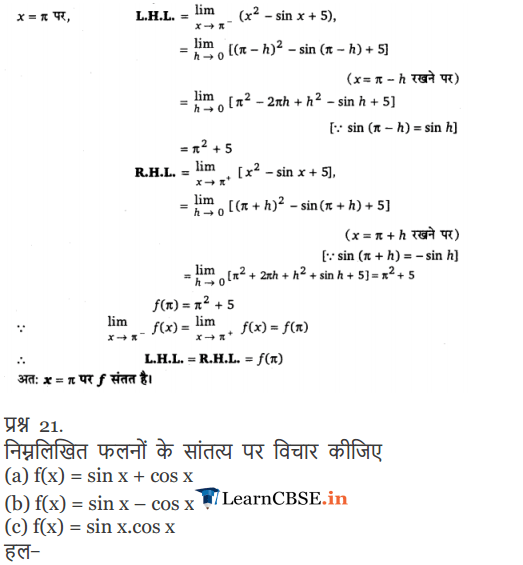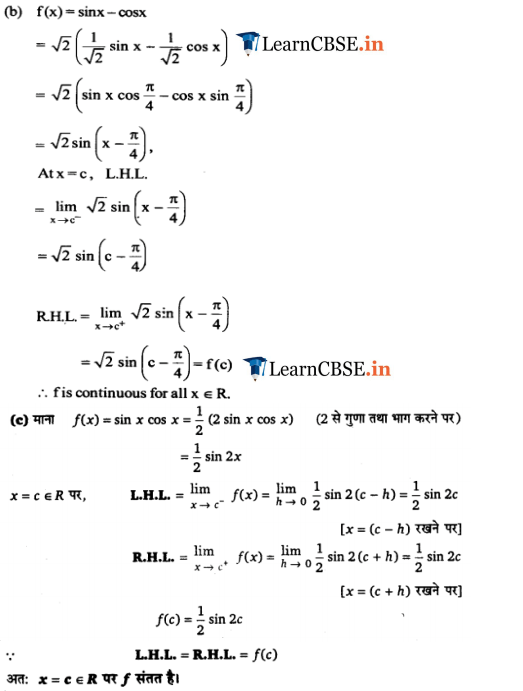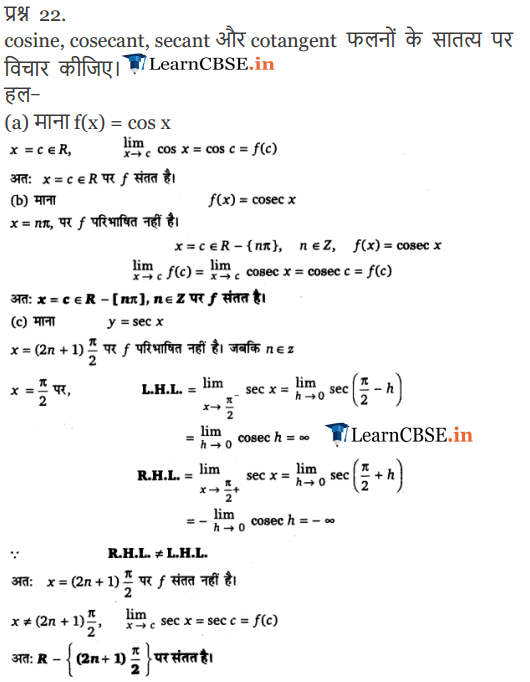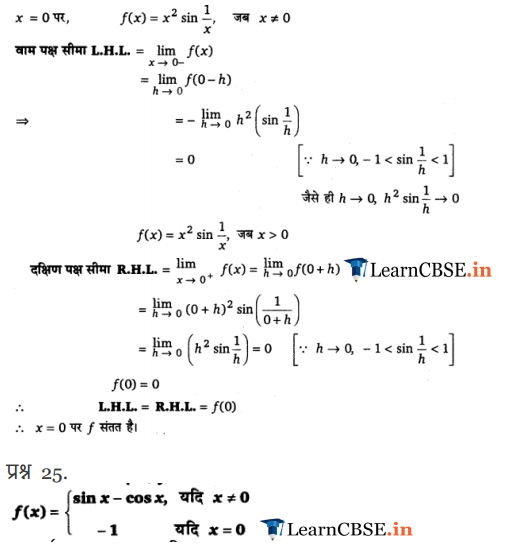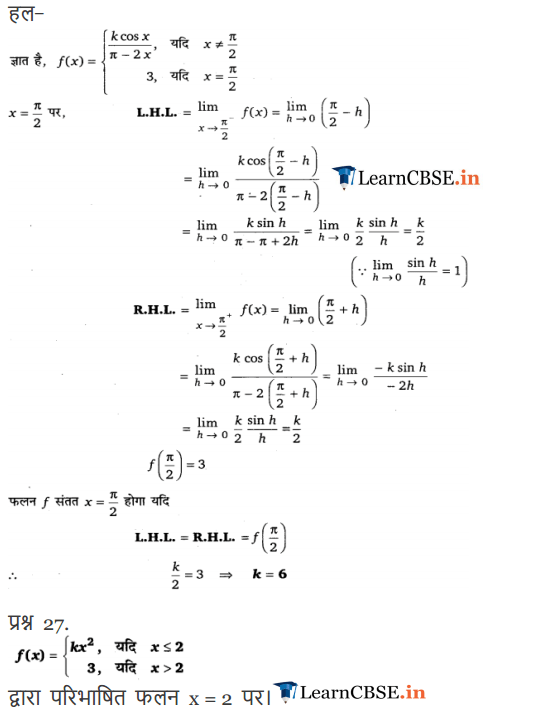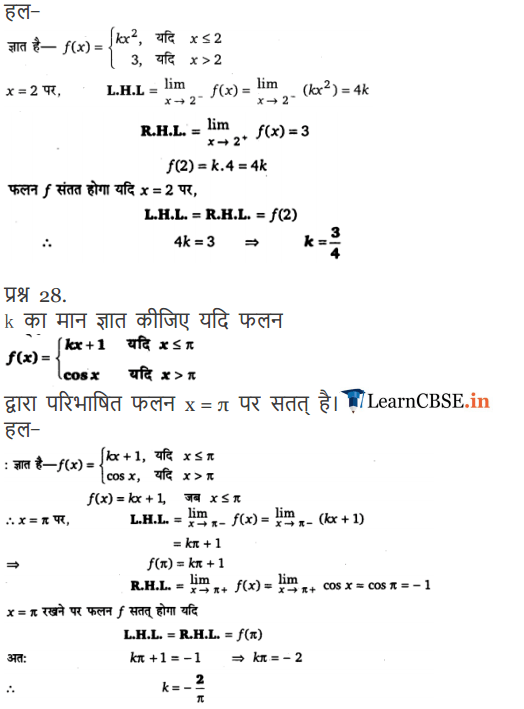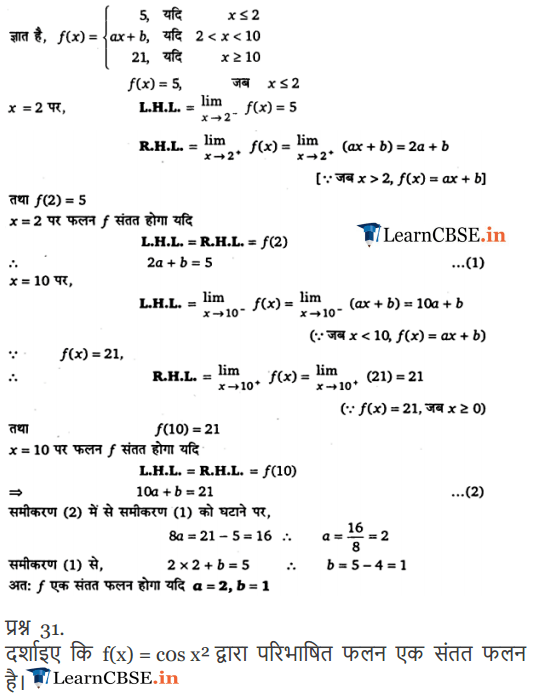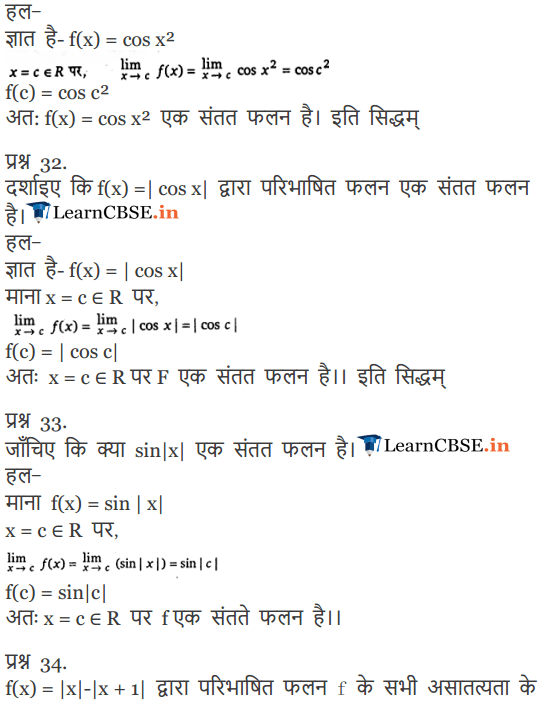# NCERT Solutions for Class 12 Maths Chapter 5 Continuity and Differentiability

NCERT Solutions for Class 12 Maths Chapter 5 Continuity and Differentiability provided here is extremely helpful in revising complete syllabus and getting a strong base on it. NCERT 12th Class Maths Continuity and Differentiability all questions are solved with detailed explanation available for students. In this article we had given of NCERT solutions for Continuity and Differentiability Class 12 Maths step by step solution for each and every question of the chapter. These solutions will also help you with your homework. Best teachers across the India created NCERT solutions for Class 12 Maths Chapter 5 Continuity and Differentiability according to curriculum and pattern of syllabus as per guidelines of NCERT (CBSE) Books.

## 12th Class NCERT Maths Chapter 5 Continuity and Differentiability

 Name of Organization NCERT Name of Class 12th Class Name of Subject Maths Name of Chapter Chapter 5 Name of Content Continuity and Differentiability Name of Category NCERT Solutions Official site http://ncert.nic.in/

### Chapter 5 Continuity and Differentiability covers numerous Questions and answers from all topics and sub-topics which are given below

The topics and sub-topics included in the Continuity and Differentiability chapter are the following:

• Continuity and Differentiability
• Introduction
• Algebra of continuous functions
• Differentiability
• Derivatives of composite functions
• Derivatives of implicit functions
• Derivatives of inverse trigonometric functions
• Exponential and Logarithmic Functions
• Logarithmic Differentiation
• Derivatives of Functions in Parametric Forms
• Second Order Derivative
• Mean Value Theorem
• Summary

### Class 12 Maths Continuity and Differentiability Exercise 5.1### Class 12 Maths Continuity and Differentiability Exercise 5.2 and 5.3### Class 12 Maths Continuity and Differentiability Exercise 5.4### Class 12 Maths Continuity and Differentiability Exercise 5.5### Class 12 Maths Continuity and Differentiability Exercise 5.6### Class 12 Maths Continuity and Differentiability Exercise 5.7### Class 12 Maths Continuity and Differentiability Exercise 5.8### Class 12 Maths Continuity and Differentiability Miscellaneous Exercise### NCERT Solutions for Class 12 Maths Chapter 5 Continuity and Differentiability Hindi Medium Ex 5.1